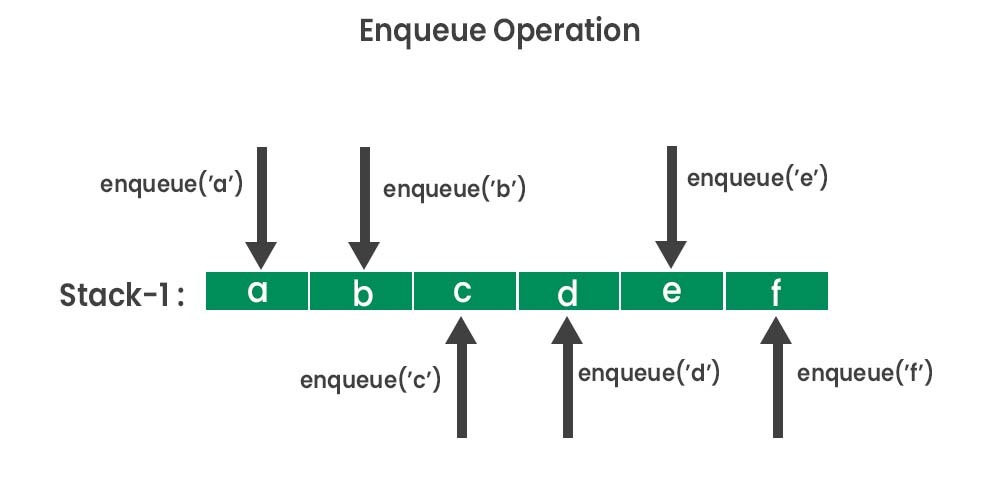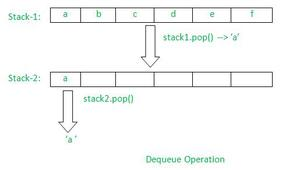GFG App
Open AppBrowser
Continue

# Implement enqueue and dequeue using only two stacks in JavaScript ?

In this article, we will implement queue’s operations (enqueue and dequeue) using only two stacks (in the form of plain arrays) in JavaScript. Before directly jumping into understanding the problem statement let us understand briefly what exactly a stack is and also what exactly a queue is.

A stack is a linear data structure that works on the concept of Last-In-First-Out (LIFO) in which the element which comes, at last, will be removed or out at first. Similarly, a queue is also a linear data structure that works on the concept of First-In-First-OUT (FIFO) in which the element which comes at first will be removed or out at first itself. Some real-life example for better understanding the concept of the stack includes: arranging plates over one another until the last plate is put on top etc. Similarly, standing in a movie ticket collecting row where one person will collect movie tickets at first and until that person leaves the row, next person will not be allowed to collect the movie tickets, is an example of a queue.

Now that you have understood briefly about Stacks and Queues, let us understand the problem statement clearly and further we will see the solution for the problem statement itself. Following pictorial representations will describe the problem statement clearly which is to implement queue operations (enqueue and dequeue) using only two stacks-The above pictorial representation represents the enqueue operation which was being performed with several elements (like a ,b, c and so on) which are inserted into stack (declared in the form of plain array).The above pictorial representation represents the dequeue operation which was being performed using several elements as well as two stacks by which we will first pop (remove) the last element from the first stack and then we will add that removed element into another stack and further remove or pop the element from the another stack. Basically removing and adding into another stack is done so that this works in reverse to what exactly stack works, or we could say reversing is done here so as to make our logic similar to queue functionality rather than working as stack itself.

Now that you have understood the problem statement very clearly let us move forward to see the approaches to solve the above illustrated problem statement.

Approach 1:

• In this approach we will first initialize two stacks (in the form of two plain arrays).
• Thereafter we will perform enqueue operation into the first stack with several elements which are given by user itself.
• Further after performing enqueue operation we will define the dequeue operation for the above inserted elements into the first stack.
• For dequeue operation we will first have to pop or remove the last element from the first stack.
• Then after removing or popping out the last element from the first stack we will add that popped element into another stack (which is also in form of array).
• Then after the adding the element into the new array we will pop or remove that element from the next stack and this way we could perform the dequeue operation.

Example:

## Javascript

 ` `

Output:

```Stack-1 elements are enqueue:  [ 'a' ]
Stack-1 elements are enqueue:  [ 'a', 'b' ]
Stack-1 elements are enqueue:  [ 'a', 'b', 'c' ]
Element after Dequeue: a
Element after Dequeue: b```

Approach 2:

• This approach works dynamically for multiple enqueue and dequeue operations.
• Here we will handle multiple cases like if dequeue was called before enqueue, or multiple dequeue’s are called, or if enqueue is just called after dequeue or only single operation is just called after dequeue.
• As similar to first approach here also we are going to make two separate functions or methods for both enqueue and dequeue operations.
• In these two separate methods we will perform the individual logics of enqueue and dequeue respectively.

Example:

## Javascript

 ` `

Output:

```Elements after Enqueue:  [ 'a' ]
Elements after Enqueue:  [ 'a', 'b' ]
Elements after Enqueue:  [ 'a', 'b', 'c' ]
Element after dequeue: a
Elements after Enqueue:  [ 'b', 'c', 'd' ]
Elements after Enqueue:  [ 'b', 'c', 'd', 'e' ]
Element after dequeue: b
Element after dequeue : c
Element after dequeue : d```

My Personal Notes arrow_drop_up